[Next]: Finite element methods for surface diffusion
 [Up]: Project descriptions
 [Previous]: Two-dimensional numerical simulation of a DMFC
[Contents]   [Index]

## Thermal convection in sedimental basins

Collaborator: J. Fuhrmann

Cooperation with: U. Bayer (GeoForschungsZentrum Potsdam)

Description:

We regard the following model of thermal convection: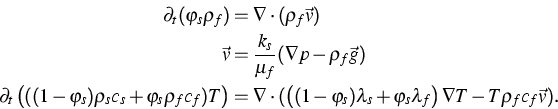These equations describe fluid flow in a saturated porous medium, and energy transport driven by thermal diffusion in both the solid matrix and the fluid, and by convection based on the fluid flux.

The basic variables are the pressure p and the temperature T.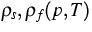, cs,cf,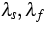are the densities, heat capacities and heat transfer coefficients for solid matrix and fluid, respectively.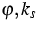are the porosity and the hydraulic permeability of the solid. The remaining data are fluid viscosity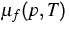, acceleration of gravity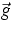and volumetric flux of water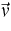. The system is closed by standard boundary conditions. When applied to sedimental basins heated from below by the Earth core, heterogeneity is introduced by the strong variation of the solid matrix parameters between the sedimental layers.

Using a time implicit, Voronoi box based finite volume method, simulations for two-dimensional slices of the North-East German basin have been carried out. These suggest that a quasi-periodic, if not chaotic flow regime might be present in the basin region.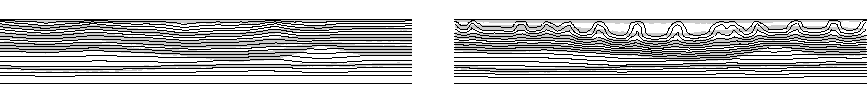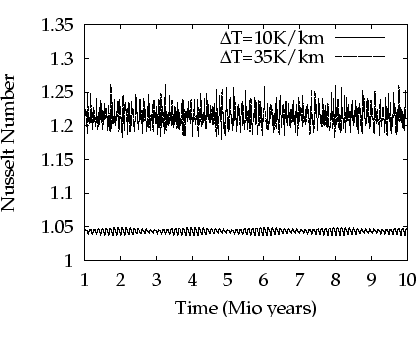From the viewpoint of the geosciences, the implications of a non-stationary flow behavior would be quite severe. Further investigation of this question, a more realistic model ansatz including the dissolved salt, and the development of three-dimensional simulation tools are subject of further research.

References:

1. U. BAYER, V. CLAUSNITZER, J. FUHRMANN, Unsteady thermal convection in the North-East-German basin, WIAS Preprint no. 741, 2002.

 [Next]: Finite element methods for surface diffusion
 [Up]: Project descriptions
 [Previous]: Two-dimensional numerical simulation of a DMFC
[Contents]   [Index]

LaTeX typesetting by I. Bremer
5/16/2003